# Transient Behavior of Capacitor

When a voltage is suddenly applied across a capacitor, which are previously uncharged, electrons shifting from source to capacitor to source start immediately. In other words, accumulation of change in the capacitor starts instantly. As the charge accumulating in the capacitor increases, the voltage developed across the capacitor increases. The voltage developed across the capacitor approaches to supply voltage the rate of charge accumulation in the capacitor decreases accordingly. When these two voltages become equal to each other there will be no more flow of charge from source to capacitor. The flows of electrons from source to capacitor and capacitor to source are nothing but electric current.

At the beginning, this current will be maximum and after certain time the current will become zero. The duration in which current changes in capacitor is known as transient period. The phenomenon of charging current or other electrical quantities like voltage, in capacitor is known as transient.
To understand transient behavior of capacitor let us draw a RC circuit as shown below,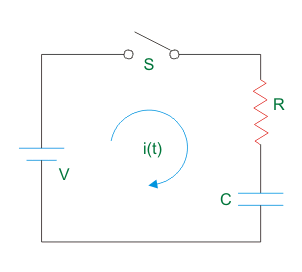Now, if the switch S is suddenly closed, the current starts flowing through the circuit. Let us current at any instant is i(t).
Also consider the voltage developed at the capacitor at that instant is Vc(t).
Hence, by applying Kirchhoff’s Voltage Law, in that circuit we get,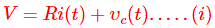Now, if transfer of charge during this period (t) is q coulomb, then i(t) can be written as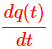Therefore,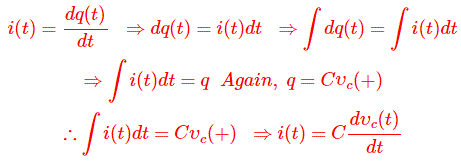Putting this expression of i(t) in equation (i) we get,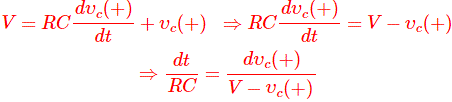Now integrating both sides with respect to time we get,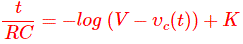Where, K is a constant can be determined from initial condition.
Let us consider the time t = 0 at the instant of switching on the circuit putting t = 0 in above equation we get,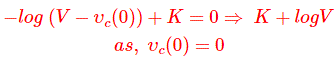There will be no voltage developed across capacitor at t = 0 as it was previously unchanged.
Therefore,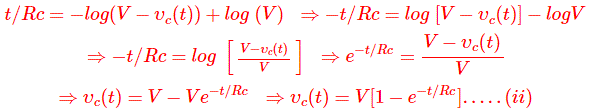Now if we put RC = t at above equation, we get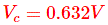This RC or product of resistance and capacitance of RC series circuit is known as time constant of the circuit. So, time constant of an RC circuit, is the time for which voltage developed or dropped across the capacitor is 63.2% of the supply voltage. This definition of time constant only holds good when the capacitor was initially unchanged.
Again, at the instant of switching on the circuit i.e. t = 0, there will be no voltage developed across the capacitor. This can also be proved from equation (ii).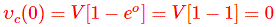So initial current through the circuit is, V/R and let us consider it as I0.
Now at any instant, current through the circuit will be,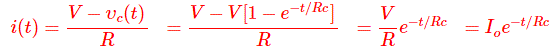Now when, t = Rc the circuit current.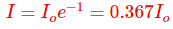So at the instant when, current through the capacitor is 36.7% of the initial current, is also known as time constant of the RC circuit.
The time constant is normally denoted will τ (taw). Hence,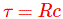## Transient During Discharging a Capacitor

Now, suppose the capacitor is fully charged, i.e. voltage at capacitor is equal to the voltage of source. Now if the voltage source is disconnected and instead two terminals of the battery are short circuited, the capacitor will stared discharging means, unequal distribution of electrons between two plates will be equalized through the short circuit path. The process of equaling electrons concentration in two plates will continue until the voltage at capacitor becomes zero. This process is known as discharging of capacitor. Now we will examine the transient behavior of capacitor during discharging.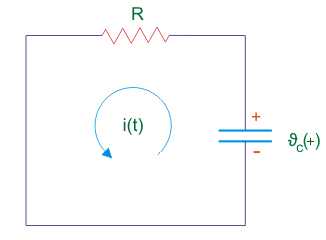Now, from the above circuit by applying Kirchhoff Current Law, we get,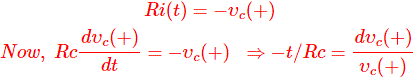Integrating both sides we get,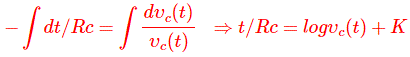K is the constant which can be determined from initial value. Now, at the time of short circuiting the capacitor,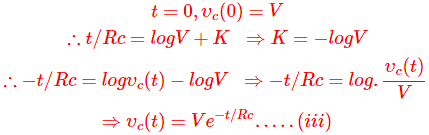Now, from equation (iii), by applying t = τ = RC we get,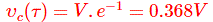Again, circuit current at that time i.e. τ = RC,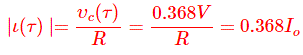Thus at time constant of capacitor, both capacitor voltage, ϑc and current i are reduced to 36.8 % of their initial value.

Want To Learn Faster? 🎓
Get electrical articles delivered to your inbox every week.
No credit card required—it’s 100% free.